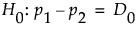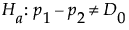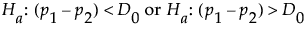Design of Experiments Guide > Prospective Sample Size and Power > Two Sample Proportions Calculator
Publication date: 08/13/2020

# Two Sample Proportions Calculator

Use the Two Sample Proportions calculator to evaluate sample size for a hypothesis test about two proportions. Sample size and power are associated with the following hypothesis test:versus the two-sided alternative:or versus either of the following one-sided alternatives:where p1 and p2 are the population proportions from two populations, and D0 is the hypothesized difference in proportions.

The Two Proportions Calculator also provides the actual test size. This is the actual Type I error rate for the specified assumptions. Since the binomial distribution is discrete, the actual test size can differ significantly from the stated Alpha level for small samples or proportions near 0 or 1.